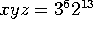M362K Second Midterm Exam
March 7, 2002

Problem 1. Political committees

13 Democrats, 12 Republicans and 8 Independents are sitting in a room. 8 of these people will be selected to serve on a special committee.

a) How many different possibilities are there for the committee membership?

b) What is the probability that exactly 5 of the committee members will be Democrats?

c) What is the probability that the committee will consist of 4 Democrats, 3 Republicans and one independent?

Problem 2. Number theory

a) How many solutions exist to the equation x+y+z = 15, where x, y, and z have to be non-negative integers? Simplify your answer as much as possible. [Note: the solution x=12, y=2, z=1 is not the same as x=1, y=2, z=12]

b) How many solutions exist to the equation, where x, y and z have to be positive integers?

Problem 3. Spring Break Drinking

On South Padre Island over spring break, 25 underage students try to buy beer with fake IDs. Each has a 10% chance of getting caught, independent of the others.

a) What is the probability that exactly 4 of the students get caught?

b) What is the probability that 3 or more students get caught?